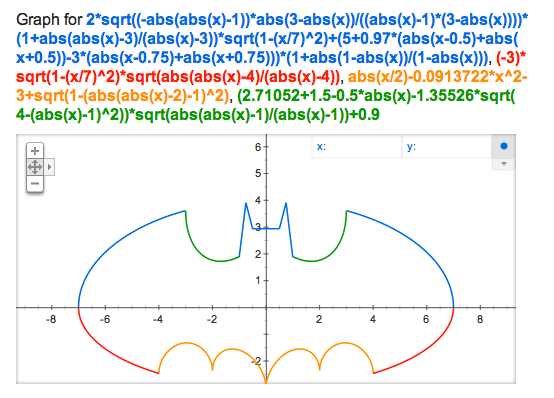Tag Archives: Batman

## Algebra Is Your Friend

16 May

Today, I accomplished something even my Honors Algebra teacher couldn’t.

I graphed Batman on my trusty TI-83, Bessie.It took me literally 45 minutes to get it right. I used three different equations before I got the desired result. If you want to give it a try:

Equation #1 (failure):

Y1 = 1.5sqrt(-abs(abs(x)-1)*abs(3-abs(x))/((a­¬bs(x)-1)*(3-abs(x))))(1+abs(abs(x)-3)/(­a¬bs(x)-3))sqrt(1-(x/7)^2)+(4.5+0.75(abs­(x¬-.5)+abs(x+.5))-2.75(abs(x-.75)+abs(x­+.7¬5)))(1+abs(1-abs(x))/(1-abs(x)))

Y2 = -3sqrt(¬1-(x/7)^2)sqrt(abs(abs(x)-4)/(ab­s(x)-4))

Y3 = abs(x/2)-0.0913722(x^2)-3+sqrt(1-(abs(a¬­bs(x)-2)-1)^2)

Y4 = (2.71052+(1.5-.5abs(x))-1¬.35526sqrt(4-(­abs(x)-1)^2))sqrt(abs(abs(¬x)-1)/(abs(x)­-1))

Yeah… there are some cowboys in that one. Number two is a bit better.

Equation #2:I like this one because it’s colorful. The different colors are different Y=’s. Go for it!

Equation #3:

Number three require you to change to “PAR” in mode. These are the parameters:

X1 = {1, -1}(2.5sin(.5T) + 4)
Y1 = .7T + 2

X2 = {1, -1}(7sin(.5T) + 4)
Y2 = 1.8T – 1.5π

X3 = {1, -1}(cos(.5T) + 1)
Y3 = 2sin(.5T) – .1T2 – 3

X4 = {1, -1}(.25T + 2)
Y4 = .5T-1 + 2

X5 = .6T – .6π
Y5 = .75sin(.5T) + 5

X6 = {1, -1}(1.4cos(.6T) – 3.4)
Y6 = 1.7sin(.6T) – 3

And that was the successful one!

I think because I did this, I shouldn’t have to to my homework tonight… eh?